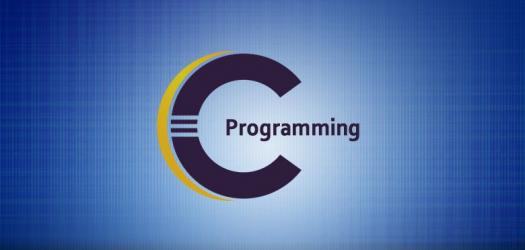# Computer Quiz: Basic Questions On C Language

10 Questions | Attempts: 923
ShareSettings.

• 1.
What will be the output of the following program? #include<stdio.h> int main() { char numbers = {"Zero","One","Two","Three","Four"}; printf("%s is %c",&numbers,numbers); return 0; }
• A.

Four is Z

• B.

Three is Z

• C.

Compiler error

• D.

Two is Z

• 2.
Which one of the following is an example of storage class in C?
• A.

Int and float

• B.

Extern

• C.

Both 1 & 2

• D.

Neither 1 nor 2

• 3.
What will be the output of the following program? #include<stdio.h> int main() { int a = 1, b = 2, c = 3; printf("The value of a is %d", ++a); func(); printf(" The value of a is %d", ++a); return 0; } void func() { int a = 10; return 0; }
• A.

The value of a is 2 The value of a is 2

• B.

The value of a is 2 The value of a is 3

• C.

The value of a is 1 The value of a is 10

• D.

Compiler error

• 4.
What  is the output of the below program? #include<stdio.h> int main() { printf("%c", "abcdefgh"); return 0; }
• A.

G

• B.

E

• C.

F

• D.

Compiler Error

• 5.
What will be the output of the following program? #include<stdio.h> #define putchar(c) printf("%c",c) int  main() { char s='c'; putchar (s); return 0; }
• A.

99

• B.

Compiler Error

• C.

Runtime Error

• D.

C

• 6.
What will be the output of the following program? #include<stdio.h> enum {TRUE, FALSE}; int main() { int i=1; do{ printf("%d\t",i); i++; if(i > 5) break; } while(TRUE); return 0; }
• A.

1 2 3 4 5

• B.

It prints an infinite loop of numbers

• C.

1 2 3 4

• D.

1

• 7.
What will be the output of the following program ? #include<stdio.h> int main(){ typedef struct{char ch;}st; st s1 = {'c'}; st s2 = s1; if(s1 == s2) printf("Successful"); return 0; }
• A.

Successful

• B.

Compiler error

• C.

Compiler warning

• D.

None of these

• 8.
What is the keyword used to turn off the compiler optimization of a variable?
• A.

Mutable

• B.

Volatile

• C.

Static

• D.

None of these

• 9.
What will be the output of the following program? #include<stdio.h> int main() { fun(); } fun() { printf("you are in fun"); main(); return 0; }
• A.

It will print "you are in fun" endlessly

• B.

Infinite loop

• C.

It is an example of tree recursion

• D.

Stack overflow

• 10.
Which one of the following is the correct way of declaring main() function when it receives command line arguments?
• A.

Main(int argc, char argv)

• B.

Main(char argv[ ], int *argc)

• C.

Main(char* argv[ ],int argc)

• D.

Main(int argc,char* argv[ ])

## Related TopicsBack to top
×

Wait!
Here's an interesting quiz for you.